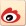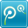# 2020年广东公务员考试数学运算指导：二次函数极值问题

2019-05-12    来源：广东公务员考试网 字号： T | T | T我要提问
【例题】某汽车租赁公司有200辆同型号的汽车，每辆车的日租金为100元时可全部租出;当每辆车的日租金增加5元时，未租出的汽车就会多4辆，租出的车每天需要维护费20元。每辆车的日租金为多少时，租赁公司的日收益最大?(  )

A. 155元

B. 165元

C. 175元

D. 185元

【解析】答案为D。假设每辆车的日租金增加x个5元，此时有最大收益y元，则根据题意可得：y=(100+5x-20)×(200-4x)，化简得y=-20x^2+680x+16000=-20[(x-17)^2-1089]，当且仅当x=17时，y最大，故所求为100+17×5=185元。故选D项。

【例题】4辆车运送货物，每辆车可运16次，7辆车运送，每辆车只能运10次。设增加的车辆数与运送减少的次数成正比，且每车次运送货物相等，运送货物总量最多是多少车次?(  )

A. 72

B. 74

C. 64

D. 68

【解析】假设x为增加的车辆数，y为总的运货车次，根据“设增加的车辆数与运送减少的次数成正比”，则有y=(4+x)×(16-2x)=-2x^2+8x-64=-2[(x-2)^2-36]，当x=2时，y有最大值，最大值是y=(4+x)×(16-2x)=(4+2)×(16-2×2)=6×12=72。故选A项。相关文章相关问题